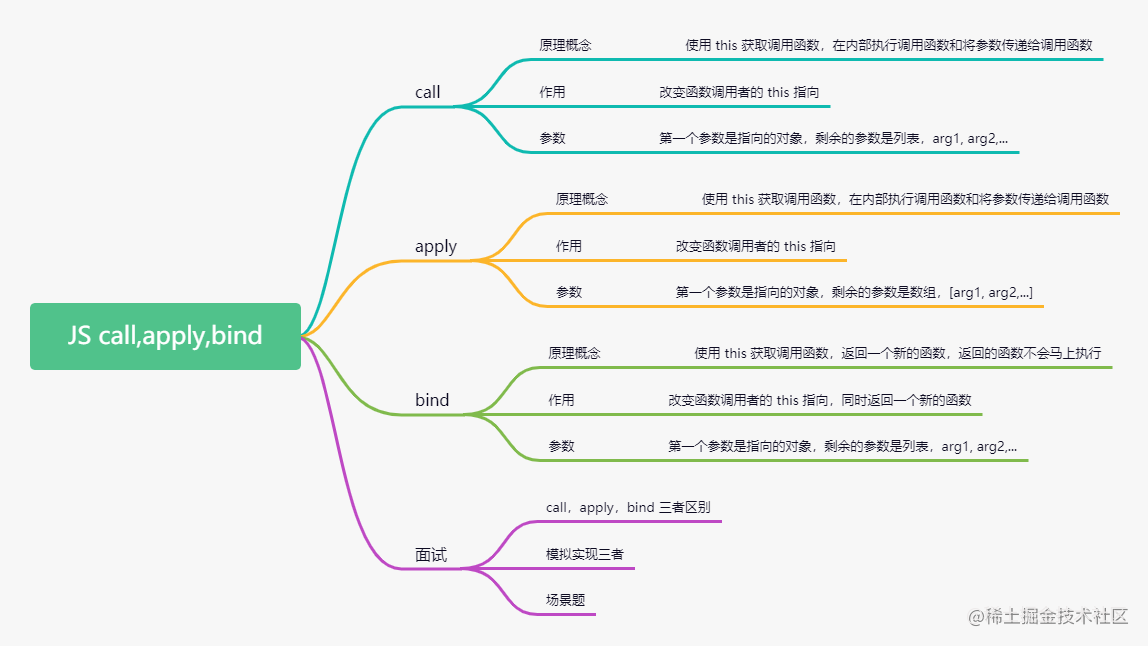# 思维导图# call，apply，bind 都可以改变 this 的指向

## 思考

### 1. 热身题1

``````function fn(a, b) {
console.log(this, a, b)
}

var obj = {
name: '林一一'
}

fn.call(obj, 20, 23)   // {name: "林一一"} 20 23

fn.call(20, 23) // Number {20} 23 undefined

fn.call()   //Window {0: global, window: …} undefined undefined     | 严格模式下为 undefined

fn.call(null)   //Window {0: global, window: …} undefined undefined       | 严格模式下为 null

fn.call(undefined)  //Window {0: global, window: …} undefined undefined     | 严格模式下为 undefined

`fn`调用了`call``fn``this` 指向 `obj`，最后 `fn` 被执行；`this` 指向的值都是引用类型，在非严格模式下，不传参数或传递 `null/undefined``this` 都指向 `window`。传递的是原始值，原始值会被包装。严格模式下，`call` 的一个参数是谁就指向谁

### 2. 热身题 2

``````var obj1 = {
a: 10,
fn: function(x) {
console.log(this.a + x)
}
}

var obj2 = {
a : 20,
fn: function(x) {
console.log(this.a - x)
}
}

obj1.fn.call(obj2, 20) //40

## 二、apply 和 call 基本一致

`两者唯一不同的是：apply 的第一个参数还是调用函数this的指向，第二个参数是数组[arg1, arg2...]，call的第二参数是列表(arg1, arg2...)`

``````var name = '二二'
var obj = {
name: '林一一',
fn: function() {
return `\${this.name + [...arguments]}`
}
}
obj.fn.apply(window, [12, 23, 34, 56])    // "二二12,23,34,56"

apply 第二个参数接收的是数组

## 面试题

### 1. 模拟实现内置的 call()，apply()方法。

• call 的模拟实现

``````Function.prototype.myCall = function (context, ...args){
context = context || window
// 这里的 this 是指向 fn 的，通过 this 就可以获取 fn，context 是我们的 obj，可以直接给 obj 添加一个函数属性
context.fn = this
context.fn(...args)
delete context.fn
return
}

var name = '二二'
var obj = {
name: '林一一',
}

function fn() {
console.log(this.name, ...arguments)
}

fn.myCall(null)
fn.myCall(obj, 12, 23, 45, 567)

• apply 的模拟实现
``````Function.prototype.myApply = function (context, args){
context = context || window
context.fn = this
context.fn(...args)
delete context.fn
return
}

### 2. call 和 apply 区别

`call` 方法的语法和作用与 `apply` 方法类似，只有一个区别，就是 call() 方法接受的是一个参数列表，而 apply() 方法接受的是一个包含多个参数的数组。

``````var name = '二二'
var obj = {
name: '林一一'
}

function fn(){
console.log(this.name, ...arguments)
}

fn.apply(obj, [12, 34, 45, 56]) //fn(12, 23, 45, 56) 林一一   12 34 45 56

## 三、bind

• 返回一个新函数，这个新函数执行时的 `this` 才指定到 `bind` 的第一个参数
• `bind` 的剩余参数，传递给新的函数
• 返回后的新函数是自我调用的，且返回的新函数还可以传入新的参数

### 1. 上面说的这个新函数是啥？

``````var name = '二二'
var obj = {
name: '林一一'
}

function fn(){
return `\${this.name} ` + [...arguments]
}

let f = fn.bind(obj, 12, 23, 45, 67, 90)
f() // "林一一 12,23,45,67,90"

## 面试题

### 2. 模拟实现内置的 bind() 方法。

• 简化版实现
``````Function.prototype.myBind = function myBind(context, ...arg) {
var _this = this    // 获取调用 myBind 的函数主体
function ctor (...otherArg){      // 返回的新函数，是调用 myBind 函数的拷贝
// 判断this的来源是不是 new 关键字的  this  instanceof  ctor 是 true，说明有实例返回，
// 也就是有使用到 new 关键字。
_this.call(this instanceof ctor ? this : context, ...arg.concat(...otherArg))          // 利用 apply 原理，改变 this 指向，同时执行返回的新函数。
}

// 修改返回函数的 prototype 为绑定函数的 prototype，实例就可以继承绑定函数的原型中的值
ctor.prototype = this.prototype
return ctor
}

var obj = {
name: '林一一'
}

function fn(){
console.log(this.name, ...arguments)
}

var a = fn.myBind(obj, 12, 23, 34, 56)
a() // 林一一 12 23 34 56

``````Function.prototype.bind2 = function (context) {

if (typeof this !== "function") {
throw new Error("Function.prototype.bind - what is trying to be bound is not callable");
}

var self = this;
var args = Array.prototype.slice.call(arguments, 1);

var fNOP = function () {};

var fBound = function () {
var bindArgs = Array.prototype.slice.call(arguments);
return self.apply(this instanceof fNOP ? this : context, args.concat(bindArgs));
}

fNOP.prototype = this.prototype;
fBound.prototype = new fNOP();
return fBound;
}

## 四、思考题

### 1. 求数组中的最大值和最小值

• 使用 Math 的 max/min 求最大最小值

``````let arr = [12, 45, 65, 3, 23, 11, 76, 8, 9, 56, 70]
let max = Math.max(...arr)  // 76
let min = Math.min(...arr)  // 3
复制代码``````
• 使用数组 sort 方法求最大最小值

``````let arr = [12, 45, 65, 3, 23, 11, 76, 8, 9, 56, 70]
let list = arr.sort(function(a, b) {
return b - a
})
let max = list   // 76
let min = list[list.length - 1] // 3
复制代码``````
• 使用 apply 求数组最大值最小值

``````let arr = [12, 45, 65, 3, 23, 11, 76, 8, 9, 56, 70]
let max = Math.max.apply(null, arr) // 76
let min = Math.min.apply(null, arr) // 3
复制代码``````

### 2. 如何判断一个数组

`Object.prototype.toString.call()``instanceof`。特别要注意 typeof 不可以判断数组类型

``````let arr = []
Object.prototype.toString.call(arr)

### 3.Object.prototype.toString.call() 为什么可以用来判断类型

``````Object.prototype.toString.call('str')   // "[object String]"
Object.prototype.toString.call(123)   // "[object Number]"
Object.prototype.toString.call({})      //  "[object Object]"
Object.prototype.toString.call([])      //  "[object Array]"

Object.prototype.toString.apply({})      //  "[object Object]"
Object.prototype.toString.apply([])      //  "[object Array]"

var f = Object.prototype.toString.bind({})
f()     //  "[object Object]"
var fn = Object.prototype.toString.bind([])
fn()   //  "[object Array]"

### 4.使用 call() 实现将类数组转化成数组

``````let array = [12, 23, 45, 65, 32]
function fn(array){
var args = [].slice.call(arguments)
return args
}
fn(array)   // [12, 23, 45, 65, 32]

## 参考

JavaScript深入之call和apply的模拟实现

JavaScript深入之bind的模拟实现

MDN bind